The aluminum electrolytic capacitor is composed of an anode foil, a cathode foil and separator paper which are wound together and impregnated with an electrolyte. The anode foil has an aluminum oxide layer acting as the dielectric. After the thin aluminum foil (65 to 100 microns) is electrochemically etched to increase the plate’s surface area, the dielectric is produced by anodic oxidation on its surface. The cathode foil, in general, utilizes no oxidation process.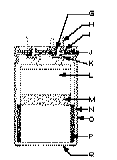A Lead Wire G Aluminum Rivet
B Aluminum Tab H Terminal
C Sealing Rubber I Rubber
D Sleeve J Phenolic
E Can K Aluminum Tab
F Element L Element
M Tape
N Can
O Sleeve
P Potting Material
Q Insulating End Disk

The Equivalent Circuit
The equivalent circuit of an aluminum electrolytic capacitor is shown below. Due to the physical design ele-ments and construction, not only does a capacitor have capacitance, but it also has a series resistance and inductance as well as a parallel resistance allowing the flow of current.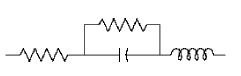RESR = Equivalent Series Resistance
R DCL = Leakage Current
C = Capacitance
L ESL = Equivalent Series Inductance

Capacitance
The capacitance of aluminum electrolytic capacitors as well as other capacitors is expressed by the following equation: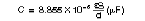Where:
E = Dielectric constant
S = Surface area of dielectric (cm2)
d = Thickness of the dielectric (cm)

Large capacitance can be obtained when:

1. the dielectric constant is high
2. the surface area is large
3. the dielectric is thin

In aluminum electrolytic capacitors the dielectric constant is only 8 to 10, but the aluminum oxide dielectric layer is extremely thin (about 15 Å per volt). High gain foil produced by the electrochemical etching creates a surface magnification, or gain, as much as 100 times for low voltage foil and 20 to 25 times for high voltage foil.

Therefore, an aluminum electrolytic capacitor can provide a large capacitance compared to other types of capacitors of the same volume.

Equivalent Series Resistance (ESR)
A critical component in the manufacturing of a capacitor that must always be considered is the equivalent series resistance. It is this resistance that leads to heat generation in the capacitor when AC current is applied. The equivalent equation for ESR is shown below.

RESR = R1 + R2 + R3

Where:

• Foil Length rl
• Tabbing
• Ohmic contact resistance

R1 = Resistance due to aluminum oxide thickness
R 2 = Resistance due to electrolyte/spacer combination
R 3 = Resistance due to materials

The amount of heat generated by ripple current depends upon the ESR of the capacitor. In order to have a low ESR, it is necessary to control the characteristics of the electrolyte, the separator paper, the winding alignment of the element, the position of the tabs and the magnification and pit construction of the etched foil. All these things contribute to the ESR of the capacitor.

Leakage Current (DCL)
The dielectric of a capacitor has a very high resistance which prevents the flow of DC current. However, there are some areas in the dielectric which allow a small amount of current to pass, called leakage current. The areas allowing current flow are due to very small foil impurity sites which are not homogeneous, and the dielectric formed over these impurities does not create a strong bond. When the capacitor is exposed to high DC voltages or high temperatures, these bonds break down and the leakage current increases. Leakage current is also determined by the following factors:

1. Capacitance value
2. Applied voltage versus rated voltage
3. Previous history

The leakage current is proportional to the capacitance and decreases as the applied voltage is reduced. If the capacitor has been at elevated temperatures without voltage applied for an extended time, some degradation of the oxide dielectric may take place which will result in a higher leakage current. Usually this damage will be repaired when voltage is reapplied.

Equivalent Series Inductance (ESL)
The inductance of a capacitor is a constant and is due primarily to the capacitor terminal spacing. The inductance runs anywhere from 4nH for miniature radial capacitors to as high as 34nH for large can capacitors. Generally speaking the inductance does not affect the overall impedance unless the capacitor is operating at extremely high frequencies.

Impedance
Impedance is the resistance which opposes the flow of alternating current at a specific frequency. It is related to capacitance and inductance in terms of capacitive and inductive reactance, and also to resistance. The impedance value is expressed in Ohms.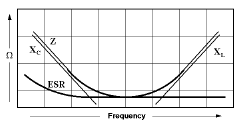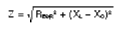Factors affecting the impedance value are:

1. Capacitive Reactance (Capacitance) – Low
Frequency Range: XC= ½(Pi)fC
2. ESR (Resistance) – Middle Frequency Range
3. Inductive Reactance (Inductance) – High
Frequency Range: XL= 2(Pi)fL

Usually, a lower impedance value in the middle frequency range is preferred. Therefore, the combination of a lower impedance value and a lower ESR value results in better capacitor performance. Due to the physical construction of a capacitor, for the same given voltage, a smaller case size capacitor has a higher ESR value. And for the same given capacitance value, a higher voltage capacitor has a lower ESR value (except for high voltage ranges).

Rated Voltage
The rated (or working) voltage is the sum of the DC voltage and the superimposed AC voltage which can be continuously applied to the capacitor. Derating the ap-plied voltage will reduce the failure rate of the capacitor.

Ripple Current
Ripple Current is the RMS value, expressed in Amps, of the alternating current flowing through a capacitor. To insure the life of aluminum electrolytic capacitors, the maximum permissible ripple current must be computed by using the following equation: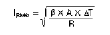Where:
T MAX = Temperature of Capacitor Center
T a = Ambient Temperature
I RMS = Ripple Current (Amps)
R = ESR (Ohms)
B = Heat Transfer Constant
A = Surface Area of Can
Delta T = Temperature Rise produced by Internal Heating (TMAX – Ta)

Where:
If the ripple current applied is higher than the specified maximum permissible ripple current computed by the equation above, the life of the capacitor becomes shorter (since the applied ripple current causes heat generation) and in extreme cases the capacitor vent will rupture. Also note that the peak voltage must not exceed the rated voltage, regardless of computed ripple current rating.